# Program to check Amicable Numbers in Java

In this tutorial you will learn about the Program to check Amicable Numbers in Java and its application with practical example.

In this tutorial, we will learn to create a Java Program to check Amicable Numbers in Java using Java programming.

## Prerequisites

Before starting with this tutorial we assume that you are best aware of the following Java programming topics:

• Java Operators.
• Basic Input and Output function in Java.
• Class and Object in Java.
• Basic Java programming.
• If-else statements in Java.
• For loop in Java.

## What is Amicable Number?

Pairs of number said to be Amicable Numbers, if sum of divisors of first number equals to the second number.
Some of the Amicable Numbers are: (220, 284), (1184, 1210), (2620, 2924), (5020, 5564), (6232, 6368) etc…

let’s have an example of one Amicable Number.
Divisors of 220 are = “1, 2, 4, 5, 10, 11, 20, 22, 44, 55, 110” on adding them we get
(1+2+4+5+10+11+20+22+44+55+110)=284
Now we will check if the Divisors of 284 = 1, 2, 4, 5, 10, 11, 20, 22, 44, 55, 110
1+2+7+71+142=220.
So we can see clearly 220 and 284 are Amicable Numbers.

## Program to check Amicable Numbers in Java

In this program , we will learn to create a Program to check Amicable Numbers.First we would declared and initialized the required variables. Next, we would prompt user to input the values. Later we will find that they are Amicable Number or not.

## Output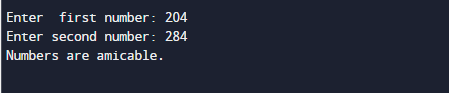In the above program, we have first declared and initialized a set variables required in the program.

• no1= it will hold first entered number.
• no2= it will hold second entered number.
• sum1=  it will calculate first divisor sum.
• sum2=  it will calculate second divisor sum.
• i = for iteration.

After that taking  values from user we will assigned the values in variable “no1” and “no2” respectively.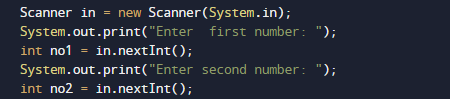Now, we have two different values in variable “no1” and  in “no2” and  now with the modulus (%) operator we find all the divisor of numbers.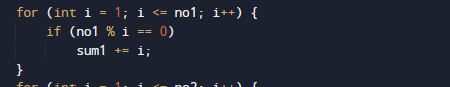and for second number.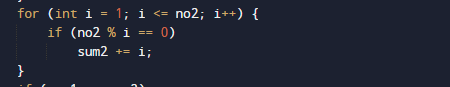## Steps for finding Amicable Number are as follow.

1. Read and initialize numbers in variables (no1, no2) from the user.
2. Find the divisor of  both the number (no1, no2) using for loop.
3. Find the sum of divisors in  variables sum1 and in sum2.
4. Checking if the sum of divisors of number no1 and no2 are equal to each other or not.

If equal, the numbers are Amicable.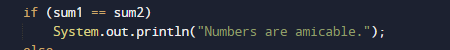Else, not Amicable.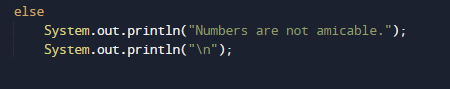So in that manner we will find the entered number are Amicable or Not.

In this tutorial we have learn about the Program to check Amicable Numbers in Java and its application with practical example. I hope you will like this tutorial.